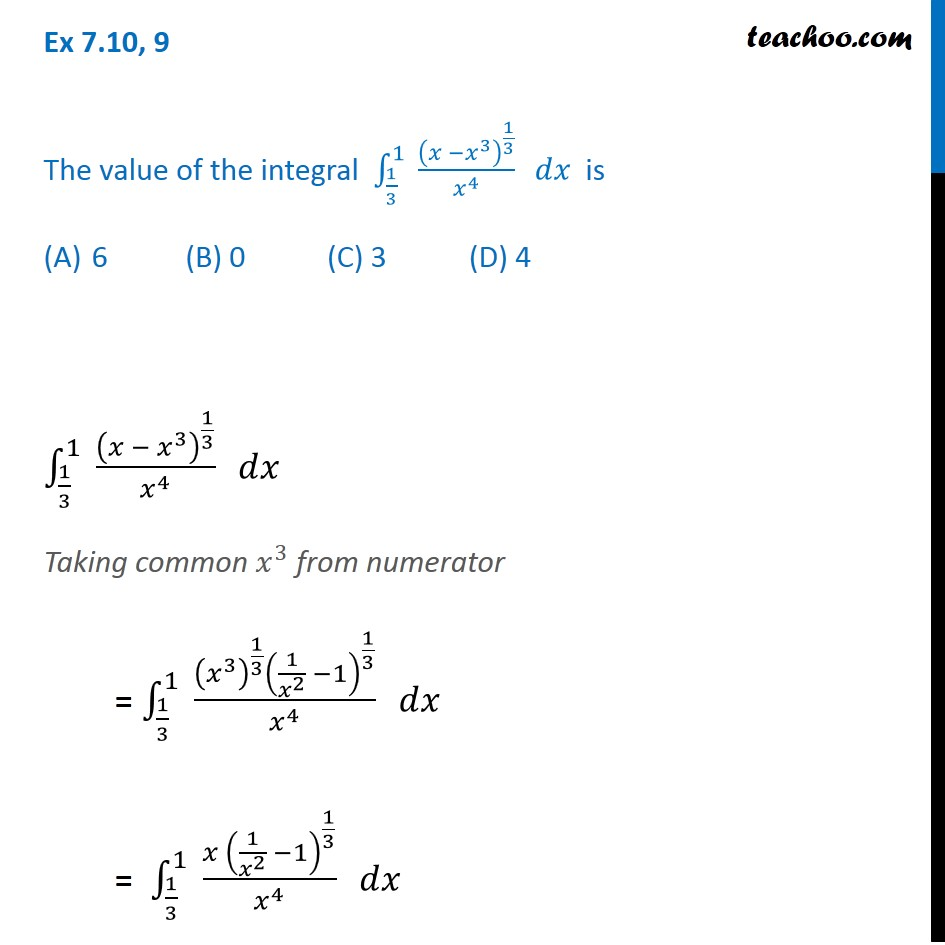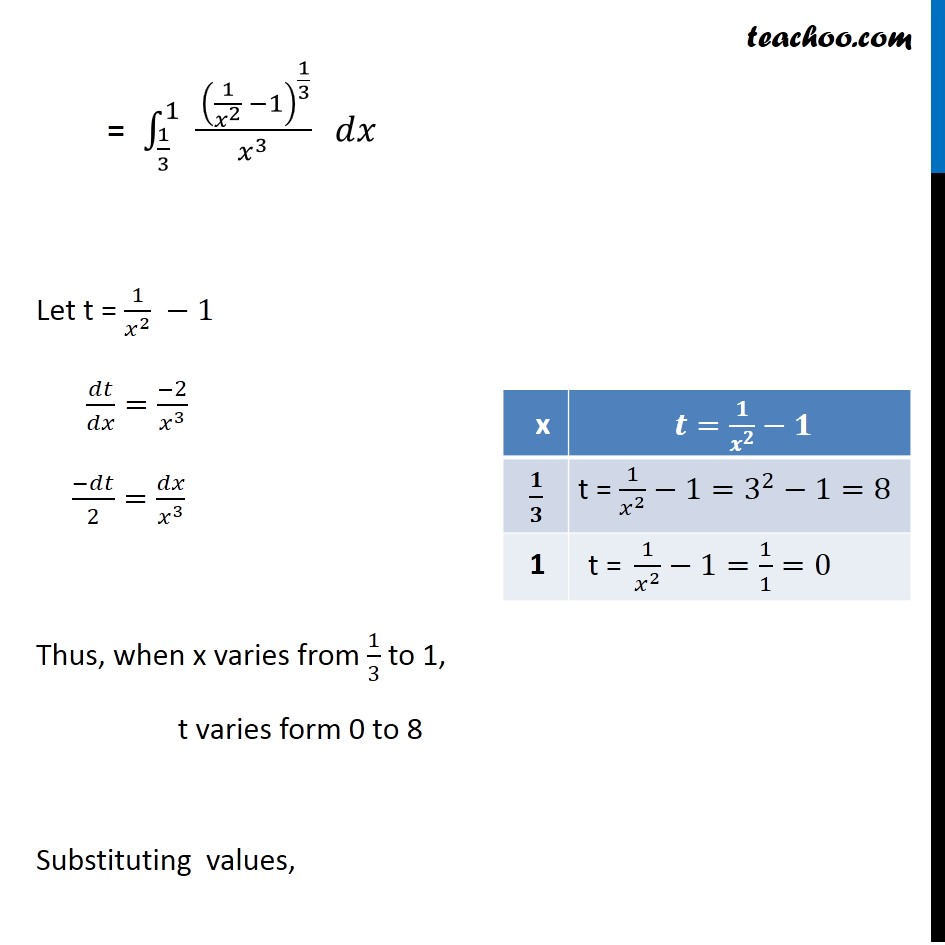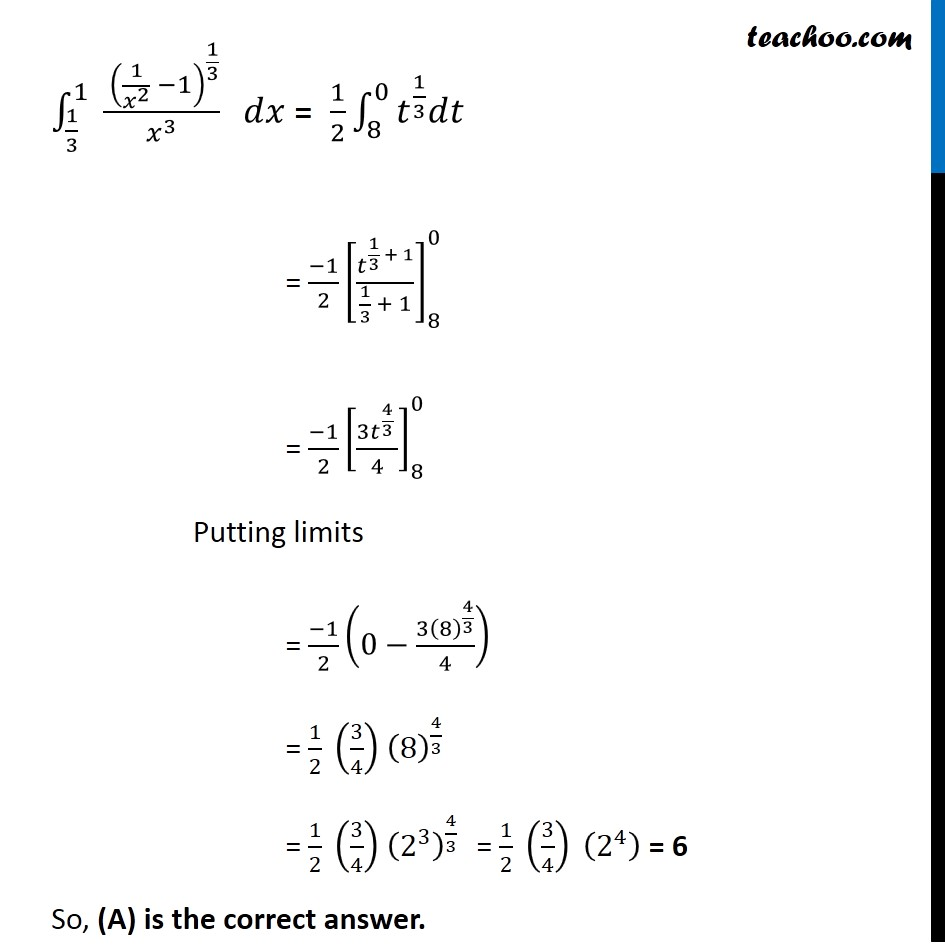Definite Integration - By Substitution

Chapter 7 Class 12 Integrals
Concept wiseIntroducing your new favourite teacher - Teachoo Black, at only ₹83 per month

### Transcript

Ex 7.10, 9 The value of the integral ∫_(1/3)^1▒〖 (𝑥 −𝑥^3 )^(1/3)/𝑥^4 〗 𝑑𝑥 is 6 (B) 0 (C) 3 (D) 4 ∫_(1/3)^1▒〖 (𝑥 − 𝑥^3 )^(1/3)/𝑥^4 〗 𝑑𝑥 Taking common 𝑥^3 from numerator = ∫_(1/3)^1▒〖 ((𝑥^3 )^(1/3) (1/𝑥^2 −1)^(1/3))/𝑥^4 〗 𝑑𝑥 = ∫_(1/3)^1▒〖 (𝑥 (1/𝑥^2 −1)^(1/3))/𝑥^4 〗 𝑑𝑥 = ∫_(1/3)^1▒〖 ( (1/𝑥^2 −1)^(1/3))/𝑥^3 〗 𝑑𝑥 Let t = 1/𝑥^2 −1 𝑑𝑡/𝑑𝑥=(−2)/𝑥^3 (−𝑑𝑡)/2=𝑑𝑥/𝑥^3 Thus, when x varies from 1/3 to 1, t varies form 0 to 8 Substituting values, ∫_(1/3)^1▒〖 ( (1/𝑥^2 −1)^(1/3))/𝑥^3 〗 𝑑𝑥 = 1/2 ∫_8^0▒〖𝑡^(1/3) 𝑑𝑡〗 = (−1)/2 [𝑡^(1/3 + 1)/(1/3 + 1)]_8^0 = (−1)/2 [〖3𝑡〗^(4/3 )/4]_8^0 Putting limits = (−1)/2 (0−(3(8)^(4/3))/4) = 1/2 (3/4) (8)^(4/3) = 1/2 (3/4) (2^3 )^(4/3) = 1/2 (3/4) (2^4 ) = 6 So, (A) is the correct answer.